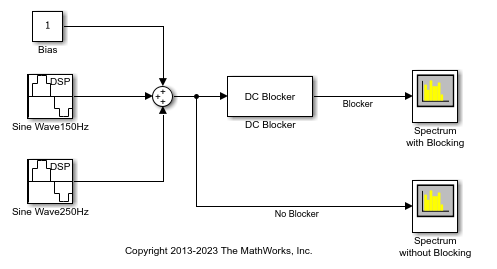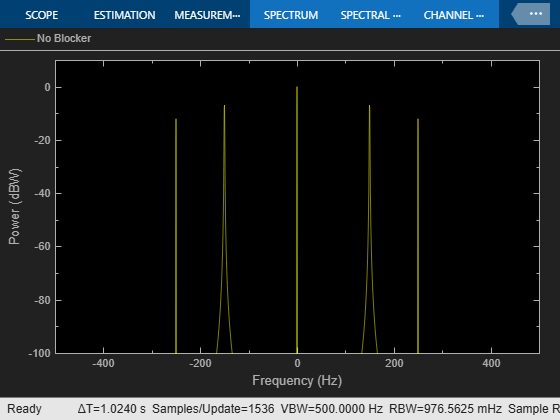# Use DC Blocker to Remove DC Component of Signal

Use the DC Blocker to remove the DC component of a signal. Use the DC Blocker first with the `IIR` algorithm and then with the `Subtract mean` algorithm to estimate the DC offset. Visualize the spectra of the input signal and the output of the DC Blocker using the Spectrum Analyzer.

The input signal contains two sinusoidal tones, one at 150 Hz and the other at 250 Hz. The two sine wave sources are set to use 1000 samples per frame because the `Subtract mean` estimation algorithm requires a statistically significant number of samples to calculate a valid mean.

Open and run the model.

The spectrum of the input signal shows tones at 150 Hz and 250 Hz and a significant (0 dBW) DC component. In the DC Blocker output, the tones at 150 Hz and 250 Hz are unaffected while the DC component has been attenuated by 30 dB.Change the estimation algorithm in DC Blocker to `Subtract mean`.

Rerun the simulation. The spectral output from the DC Blocker shows that the Subtract mean estimation method results in a DC component of less than -100 dBW.Try all three estimation methods. Modify the IIR and FIR parameters to illustrate the performance of the DC Blocker using the various estimation techniques.